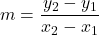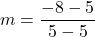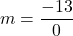## What is an equation of the line that passes through the points (5, 5) and (5, -8)

Question

What is an equation of the line that passes through the points (5, 5) and (5, -8)

in progress 0
3 weeks 2021-08-22T18:28:20+00:00 2 Answers 1 views 0

Write the problem as a mathematical expression. (5,5), (5,−8)

Use y=mx+b to calculate the equation of the line, where m represents the slope and b represents the y-intercept. To calculate the equation of the line, use the y=mx+b format.

Slope is equal to the change in y over the change in x , or rise over run.

m=(change in y)/(change in x)

The change in x is equal to the difference in x-coordinates (also called run), and the change in y

is equal to the difference in y-coordinates (also called rise).

m=(y2−y1) / (x2−x1)

Substitute in the values of x and y

into the equation to find the slope.

m=(−8−(5)) / (5−(5))

Finding the slope m.

Undefined

x=5

Step-by-step explanation:

m = undefined

General Formulas and Concepts:

Pre-Algebra

Order of Operations: BPEMDAS

1. Brackets
2. Parenthesis
3. Exponents
4. Multiplication
5. Division
7. Subtraction
• Left to Right

Algebra I

• Coordinates (x, y)
• Slope Formula:Step-by-step explanation:

Step 1: Define

Point (5, 5)

Point (5, -8)

Step 2: Identify

(5, 5) → x₁ = 5, y₁ = 5

(5, -8) → x₂ = 5, y₂ = -8

Step 3: Find slope m

Simply plug in the 2 coordinates into the slope formula to find slope m

1. Substitute in points [Slope Formula]:2. [Slope] Subtract:Here we see that we have a number divided by 0. Since we know that we cannot divide any number by 0, our slope will be undefined. This will be a vertical line on a Cartesian plane of x = 5.